# Unit Circle Flashcards

Unit Circle Flashcards
1/66 (missed) 0 0
Create Your Account To Continue Studying

As a member, you'll also get unlimited access to over 79,000 lessons in math, English, science, history, and more. Plus, get practice tests, quizzes, and personalized coaching to help you succeed.

Try it risk-free for 30 days. Cancel anytime
Use your unit circle knowledge to find: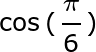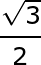Got it
Use your unit circle knowledge to find: sin (0)

0

Got it
Use your unit circle knowledge to find: cos (0o)

1

Got it
Give the degree measure of the following: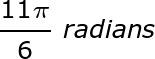330o

Got it
Give the degree measure of the following: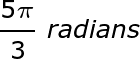300o

Got it
Give the degree measure of the following: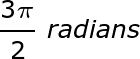270o

Got it
Give the degree measure of the following: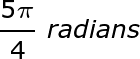225o

Got it
Give the degree measure of the following: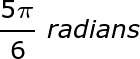150o

Got it
Give the degree measure of the following: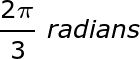120o

Got it
Give the degree measure of the following: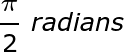90o

Got it
Give the degree measure of the following: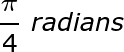45o

Got it
Give the radian measure of the following: 315o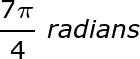Got it
Give the radian measure of the following: 240o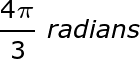Got it
Give the radian measure of the following: 210o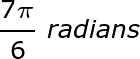Got it
Give the radian measure of the following: 180o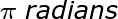Got it
Give the radian measure of the following: 135o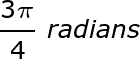Got it
Give the radian measure of the following: 60o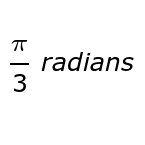Got it
Give the radian measure of the following: 30o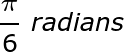Got it
Give the radian measure of the following: 0o

Got it
Unit Circle

A circle with a radius of one.

Got it

or choose a specific lesson: See all lessons in this chapter
66 cards in set

The unit circle is one of the most helpful tools in trigonometry. Memorizing the reference angles along with their corresponding ordered pairs can help a student quickly determine the sine or cosine of the angle measure. For the unit circle to be truly helpful however, a student must be able to bounce back and forth from degrees to radians for each of the 16 reference angles. You will find cards testing this skill along with ordered pair identification and trig questions involving sine and cosine of the reference angles in both units. We suggest you use this set to test yourself for not only accuracy but speed as well. For when you can answer these questions with both, you will get the most out of this handy mathematical tool.

Front
Back
Unit Circle

A circle with a radius of one.

Give the radian measure of the following: 0o

Give the radian measure of the following: 30oGive the radian measure of the following: 60oGive the radian measure of the following: 135oGive the radian measure of the following: 180oGive the radian measure of the following: 210oGive the radian measure of the following: 240oGive the radian measure of the following: 315oGive the degree measure of the following:45o

Give the degree measure of the following:90o

Give the degree measure of the following:120o

Give the degree measure of the following:150o

Give the degree measure of the following:225o

Give the degree measure of the following:270o

Give the degree measure of the following:300o

Give the degree measure of the following:330o

Use your unit circle knowledge to find: cos (0o)

1

Use your unit circle knowledge to find: sin (0)

0

Use your unit circle knowledge to find:Use your unit circle knowledge to find: sin (30o)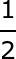Use your unit circle knowledge to find: cos (45o)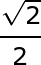Use your unit circle knowledge to find: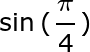Use your unit circle knowledge to find: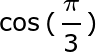Use your unit circle knowledge to find: sin (60o)Use your unit circle knowledge to find: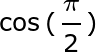0

Use your unit circle knowledge to find: sin (90o)

1

Use your unit circle knowledge to find: cos (120o)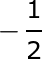Use your unit circle knowledge to find: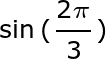Use your unit circle knowledge to find: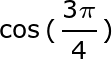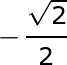Use your unit circle knowledge to find: sin (135o)Use your unit circle knowledge to find: cos (150o)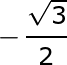Use your unit circle knowledge to find: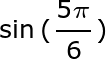Use your unit circle knowledge to find: cos (180o)

-1

Use your unit circle knowledge to find: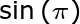0

Use your unit circle knowledge to find: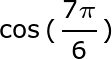Use your unit circle knowledge to find: sin (210o)Use your unit circle knowledge to find: cos (225o)Use your unit circle knowledge to find: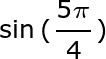Use your unit circle knowledge to find: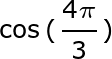Use your unit circle knowledge to find: sin (240o)Use your unit circle knowledge to find: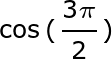0

Use your unit circle knowledge to find: sin (270o)

-1

Use your unit circle knowledge to find: cos (300o)Use your unit circle knowledge to find: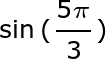Use your unit circle knowledge to find: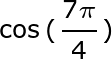Use your unit circle knowledge to find: sin (315o)Use your unit circle knowledge to find: cos (330o)Use your unit circle knowledge to find: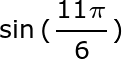Reference Angles

These are given in both radians and degrees on the unit circle. They are the only angles which have trigonometry function answers that can be written in fraction form.

Give the ordered pair for the point shown: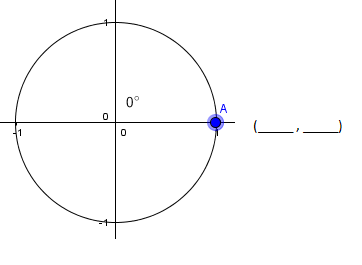(1, 0)

Give the ordered pair of the point shown: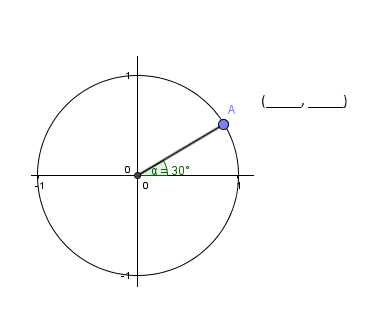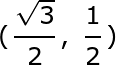Give the ordered pair of the point shown: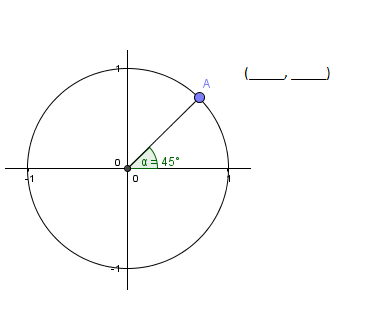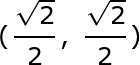Give the ordered pair of the point shown: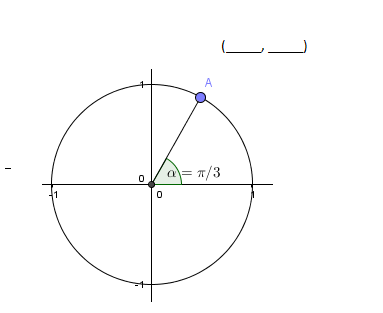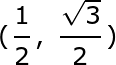Give the ordered pair of the point shown: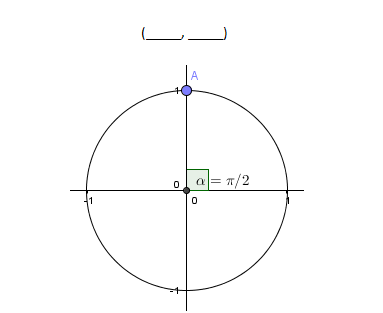(0, 1)

Give the ordered pair of the point shown: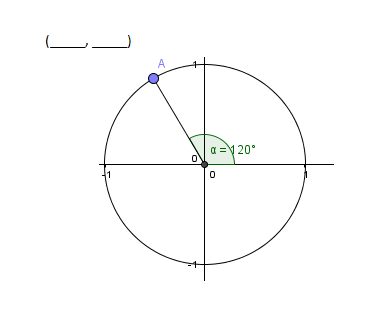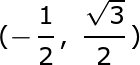Give the ordered pair of the point shown: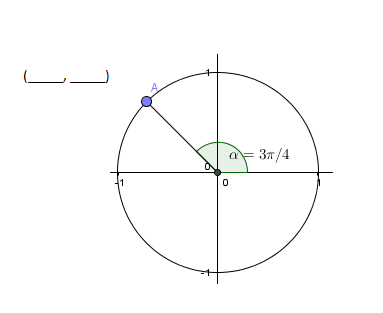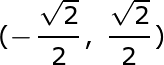Give the ordered pair of the point shown: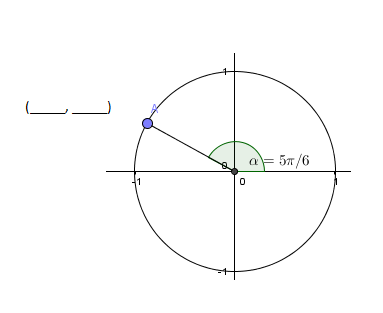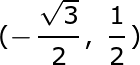Give the ordered pair of the point shown:(-1, 0)

Give the ordered pair of the point shown: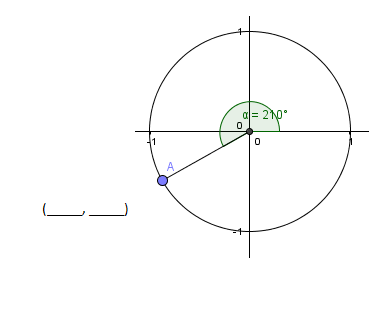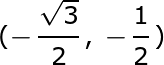Give the ordered pair of the point shown: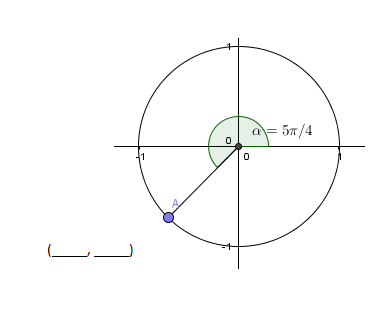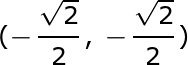Give the ordered pair of the point shown: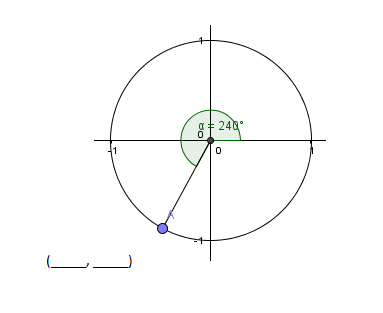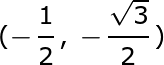Give the ordered pair of the point shown: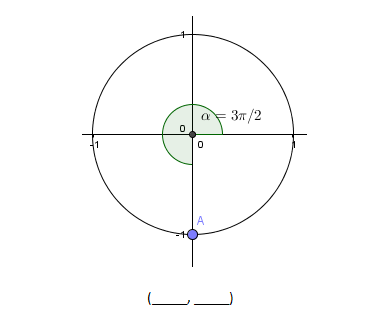(0, -1)

Give the ordered pair of the point shown: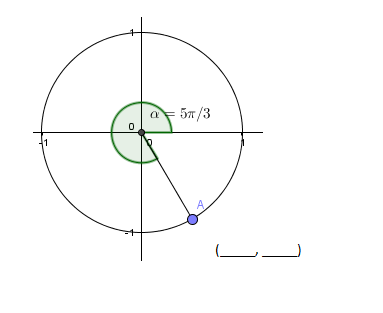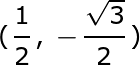Give the ordered pair of the point shown: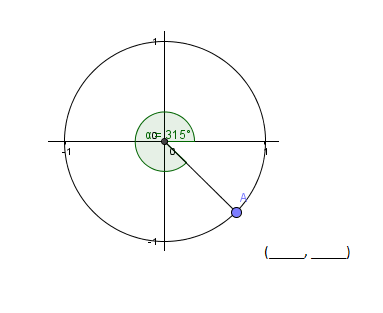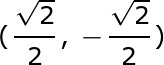Give the ordered pair of the point shown: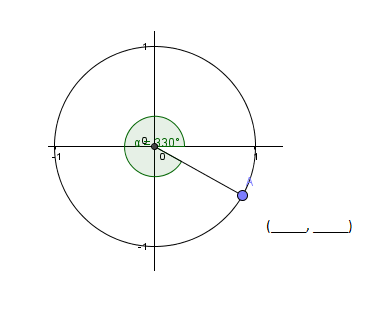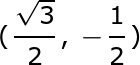To unlock this flashcard set you must be a Study.com Member.